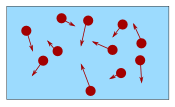# Conversion of Temperature

### Quick Celsius (°C) / Fahrenheit (°F) Conversion:

measure/images/thermometer.js?mode=boxes

Type a value in either box

Or use the slider

Or the Interactive Thermometer

Or this method:

 °C to °F Divide by 5, then multiply by 9, then add 32 °F to °C Deduct 32, then multiply by 5, then divide by 9

## Typical Temperatures

(only bold are exactly the same)

°C °F Description 220 430 Hot Oven 180 360 Moderate Oven 100 212 Water boils 40 104 Hot Bath 37 98.6 Body temperature 30 86 Beach weather 21 70 Room temperature 10 50 Cool Day 0 32 Freezing point of water −18 0 Very Cold Day −40 −40 Extremely Cold Day (and the same number!)## Some Tricks:

Daily Temperatures: these three conversions "flip the digits" (accurate within 1°):

°C   °F
28 ⇄ 82
16 ⇄ 61
04 ⇄ 40

Oven Temperatures: in the range 150 to 200 °C we can double °C to get °F (accurate within 8°F):

 °C °FEstimate °FActual 200 400 392 180 360 356 160 320 320 150 300 302

Going the other way: for the range 300 to 400 °F we can halve °F to get °C (accurate within 4°C).

## Explanation

There are two main temperature scales:

• °C, the Celsius Scale (part of the Metric System, used in most countries)
• °F, the Fahrenheit Scale (used in the US)

They both measure the same thing (temperature!), but use different numbers:

• Boiling water (at normal pressure) measures 100° in Celsius, but 212° in Fahrenheit
• And as water freezes it measures 0° in Celsius, but 32° in Fahrenheit

Like this:Looking at the diagram, notice:

• The scales start at a different number (0 vs 32), so we will need to add or subtract 32
• The scales rise at a different rate (100 vs 180), so we will also need to multiply

And so, to convert:

from Celsius to Fahrenheit: first multiply by 180100, then add 32
from Fahrenheit to Celsius: first subtract 32, then multiply by 100180

180100 can be simplified to 95, and 100180 can be simplified to 59, so we get this:

°C to °F:   Divide by 5, then multiply by 9, then add 32

°F to °C:   Subtract 32, then multiply by 5, then divide by 9

### Example: Convert 25° Celsius (a nice warm day) to Fahrenheit

First: 25° / 5 = 5
Then: 5 × 9 = 45
Then: 45 + 32 = 77° F

### Example: Convert 98.6° Fahrenheit (normal body temperature) to Celsius

First: 98.6° − 32 = 66.6
Then: 66.6 × 5 = 333
Then: 333 / 9 = 37° C

We can swap the order of divide and multiply if we want, but don't change the add or subtract. So this is also OK:

### Example: Convert 98.6° Fahrenheit to Celsius (again)

First: 98.6° − 32 = 66.6
Then: 66.6 / 9 = 7.4
Then: 7.4 × 5 = 37° C

(Same answer as before, was it easier or harder this way?)

We can write them as formulas:
Celsius to Fahrenheit:   (°C × 95) + 32 = °F
Fahrenheit to Celsius:   (°F − 32) × 59 = °C

## Other Methods That Work

### Use 1.8 instead of 9/5

9/5 is equal to 1.8, so we can also use this method:

Celsius to Fahrenheit:   °C × 1.8 + 32 = °F
Fahrenheit to Celsius:   (°F − 32) / 1.8 = °C

To make "×1.8" easier we can multiply by 2 and subtract 10%, but it only works for °C to °F:

Celsius to Fahrenheit:   (°C × 2) less 10% + 32 = °F

### Example: Convert 20° Celsius (A nice day) to Fahrenheit

• 20x2 = 40
• less 10% is 40−4 = 36
• 36+32 = 68° F

### Add 40, Multiply, Subtract 40

Since both scales cross at −40° (−40° C equals −40° F) we can:

• multiply by 5/9 (for °F to °C), or 9/5 (for °C to °F)
• subtract 40

Like this:

Celsius to Fahrenheit:   Add 40, multiply by 9/5, then subtract 40
Fahrenheit to Celsius:   Add 40, multiply by 5/9, then subtract 40

### Example: Convert 10° Celsius (A cool day) to Fahrenheit

• 10+40 = 50
• 50×9/5 = 90
• 90−40 = 50° F

### Quick, but Not Accurate

Celsius to Fahrenheit:   Double, then add 30
Fahrenheit to Celsius:   Subtract 30, then halve

Examples °C → °F:

• 0° C → 0+30 → 30° F (low by 2°)
• 10° C → 20+30 → 50° F (exact!)
• 30° C → 60+30 → 90° F (high by 4°)
• 180° C → 360+30 → 390° F (high by 34°, not good)

Examples °F → °C:

• 40° F → 10/2 → 5° C (almost right)
• 80° F → 50/2 → 25° C (low by about 2°)
• 120° F → 90/2 → 45° C (low by about 4°)
• 450° F → 420/2 → 210° C (low by about 22°, not good)Footnote: Temperature is a measure of how fast an object's particles are moving.

1041, 1042, 1043, 1044, 3724, 3725, 3726, 3727, 3728, 3729